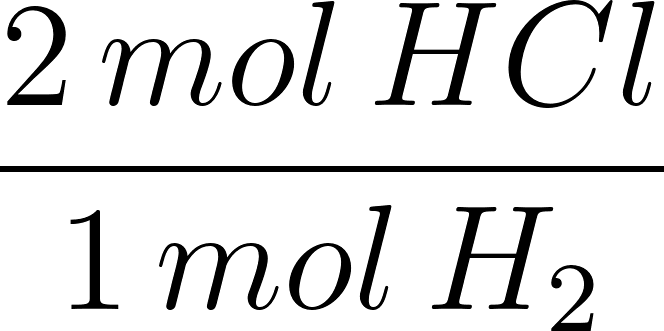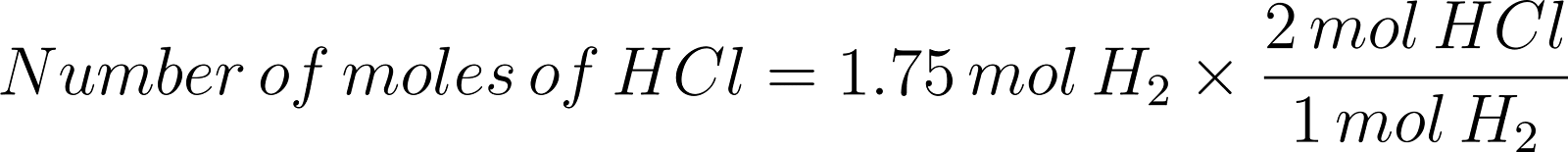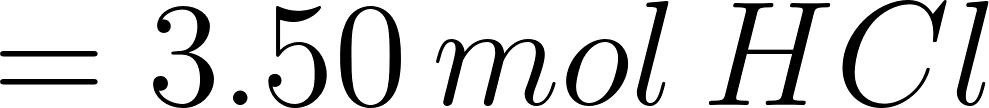×
Get Full Access to Introductory Chemistry - 5 Edition - Chapter 8 - Problem 21p
Get Full Access to Introductory Chemistry - 5 Edition - Chapter 8 - Problem 21p

×

# Solved: For each reaction, calculate how many moles of product form when 1.75 mol of theISBN: 9780321910295 34

## Solution for problem 21P Chapter 8

Introductory Chemistry | 5th Edition

• Textbook Solutions
• 2901 Step-by-step solutions solved by professors and subject experts
• Get 24/7 help from StudySoup virtual teaching assistantsIntroductory Chemistry | 5th Edition

4 5 1 409 Reviews
26
0
Problem 21P

PROBLEM 21P

For each reaction, calculate how many moles of product form when 1.75 mol of the reactant in color completely reacts. Assume there is more than enough of the other reactant.

(a) H2(g) + Cl2(g) → 2HCl(g)

(b) 2 H2(g) + O2(g) → 2 H2O(l)

(c) 2 Na(s) + O2(g) → Na2O2(s)

(d) 2 S(s) + 3 O2(g) →2 SO3(g)

Step-by-Step Solution:

Problem 21P

For each reaction, calculate how many moles of product form when 1.75 mol of the reactant in color completely reacts. Assume there is more than enough of the other reactant.

(a) H2(g) + Cl2(g) ? 2HCl(g)

(b) 2 H2(g) + O2(g) ? 2 H2O(l)

(c) 2 Na(s) + O2(g) ? Na2O2(s)

(d) 2 S(s) + 3 O2(g) ?2 SO3(g)

Step by step solution

Step 1 of 4

(a)

The given chemical reaction is as follows.From the given reaction,

One mole of hydrogen produces two moles of HCl.

The conversion factor will be ;Number of moles of hydrogen participated in the reaction = 1.75 mol

Let’s calculate the number of moles of HCl produced by using 1.75 mol of hydrogen.Therefore, the number of moles of HCl produced from 1.75 moles ofis 3.50 mol HCl.

___________________________________________________________________________

Step 2 of 4

Step 3 of 4

##### ISBN: 9780321910295

The answer to “For each reaction, calculate how many moles of product form when 1.75 mol of the reactant in color completely reacts. Assume there is more than enough of the other reactant.(a) H2(g) + Cl2(g) ? 2HCl(g)(b) 2 H2(g) + O2(g) ? 2 H2O(l)(c) 2 Na(s) + O2(g) ? Na2O2(s)(d) 2 S(s) + 3 O2(g) ?2 SO3(g)” is broken down into a number of easy to follow steps, and 55 words. The full step-by-step solution to problem: 21P from chapter: 8 was answered by , our top Chemistry solution expert on 05/06/17, 06:45PM. This textbook survival guide was created for the textbook: Introductory Chemistry, edition: 5. Since the solution to 21P from 8 chapter was answered, more than 1563 students have viewed the full step-by-step answer. This full solution covers the following key subjects: reactant, mol, color, completely, enough. This expansive textbook survival guide covers 19 chapters, and 2046 solutions. Introductory Chemistry was written by and is associated to the ISBN: 9780321910295.

## Discover and learn what students are asking

Calculus: Early Transcendental Functions : Inverse Trigonometric Functions: Integration
?In Exercises 1-20, find the indefinite integral. $$\int \frac{1}{x \sqrt{4 x^{2}-1}} d x$$

Calculus: Early Transcendental Functions : Differential Equations: Growth and Decay
?In Exercises 1-10, solve the differential equation. $$\frac{d y}{d x}=y+3$$

Calculus: Early Transcendental Functions : Integration by Parts
?In Exercises 49-54, use the tabular method to find the integral. $$\int x^{2} e^{2 x} d x$$

Unlock Textbook Solution